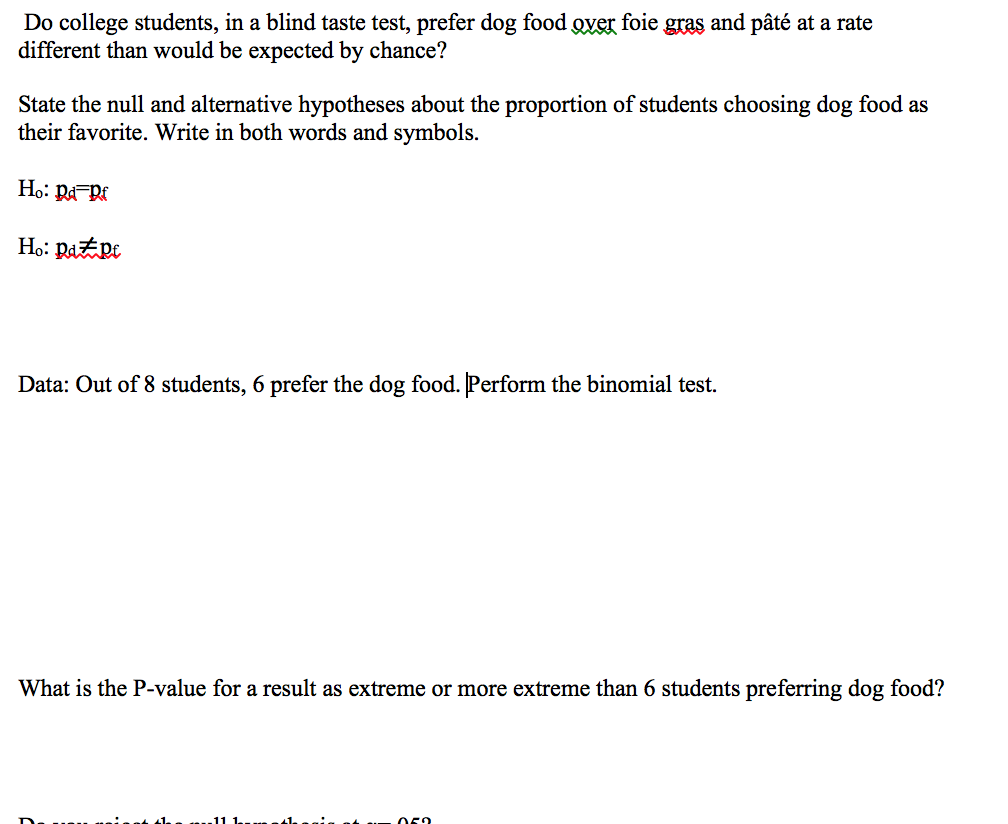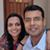D

#### Statistic

36 viewed last edited 5 years agoAnonymous
0Which Equations to use1

The Null Hypothesis would be that students are equally disposed towards dog food and fois gras. The Hypothesis would be that students prefer dog food to fois gras

Given the Null Hypothesis, the probability of at least 6 students preferring dog food is (8c8 + 8c7 + 8c6) * 2^-8 = (1+8+28) * (2^-8) = 0.14453125

Since this probability is > 0.05, we cannot reject the null hypothesis at this level of significanceMahesh Godavarti
0

Let's say the hypothesis you are testing is that the student body is atypical and prefers dog food. The null hypothesis is that the student body is a typical student body.

Let p be the probability that a student typically prefers dog food. And let q = 1 - p be the probability that a student does not.

Let's say out of N students, at least M prefer the dog food. The probability of that happening is P=\sum_{k=M}^N\binom{N}{k}\ p^kq^{N-k}.

If you set your significance as 5\% and P \gt 0.05 then you reject the null hypothesis that this is a typical population.